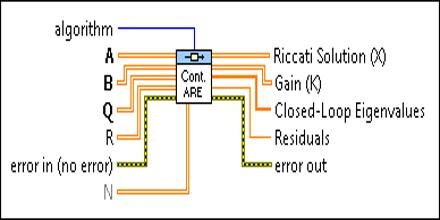Mathematic

# Algebraic Riccati EquationThis article describe about Algebraic Riccati Equation, which is not restrictive for Riccati equations arising in control of infinite-dimensional systems. It is a type of nonlinear equation that arises in the context of infinite-horizon optimal control problems in continuous time or discrete time. It is verified by the time invariant solutions of the matrix valued Riccati difference equation, which is the analogue of the Riccati differential equation in the context of discrete time LQR. Algebraic Riccati Equation determines the solution of the infinite-horizon time-invariant Linear-Quadratic Regulator problem (LQR) as well as that of the infinite horizon time-invariant Linear-Quadratic-Gaussian control problem (LQG).